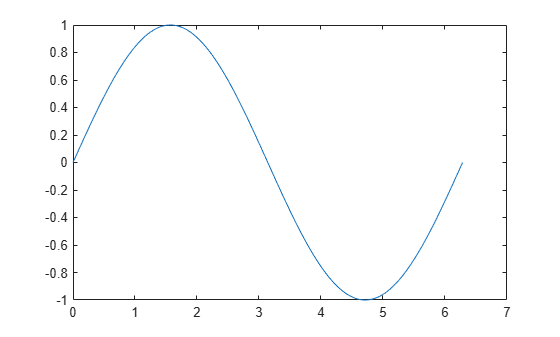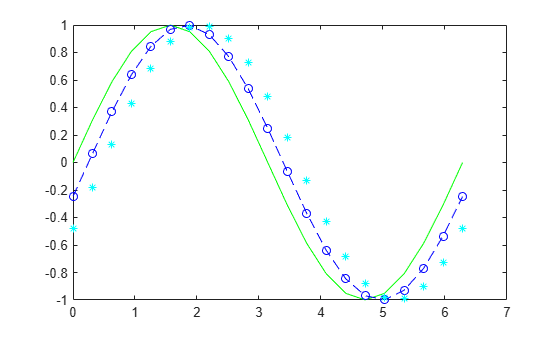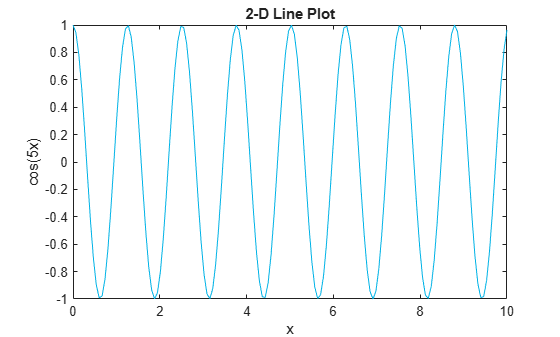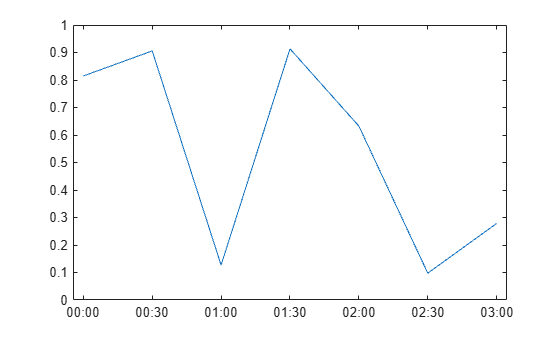# plot

## Syntax

``plot(X,Y)``
``plot(X,Y,LineSpec)``
``plot(X1,Y1,...,Xn,Yn)``
``plot(X1,Y1,LineSpec1,...,Xn,Yn,LineSpecn)``
``plot(Y)``
``plot(Y,LineSpec)``
``plot(___,Name,Value)``
``plot(ax,___)``
``h = plot(___)``

## Description

example

````plot(X,Y)` creates a 2-D line plot of the data in `Y` versus the corresponding values in `X`. If `X` and `Y` are both vectors, then they must have equal length. The `plot` function plots `Y` versus `X`.If `X` and `Y` are both matrices, then they must have equal size. The `plot` function plots columns of `Y` versus columns of `X`.If one of `X` or `Y` is a vector and the other is a matrix, then the matrix must have dimensions such that one of its dimensions equals the vector length. If the number of matrix rows equals the vector length, then the `plot` function plots each matrix column versus the vector. If the number of matrix columns equals the vector length, then the function plots each matrix row versus the vector. If the matrix is square, then the function plots each column versus the vector.If one of `X` or `Y` is a scalar and the other is either a scalar or a vector, then the `plot` function plots discrete points. However, to see the points you must specify a marker symbol, for example, `plot(X,Y,'o')`. ```
````plot(X,Y,LineSpec)` sets the line style, marker symbol, and color. ```

example

````plot(X1,Y1,...,Xn,Yn)` plots multiple `X`, `Y` pairs using the same axes for all lines. ```

example

````plot(X1,Y1,LineSpec1,...,Xn,Yn,LineSpecn)` sets the line style, marker type, and color for each line. You can mix `X`, `Y`, `LineSpec` triplets with `X`, `Y` pairs. For example, `plot(X1,Y1,X2,Y2,LineSpec2,X3,Y3)`.```

example

````plot(Y)` creates a 2-D line plot of the data in `Y` versus the index of each value. If `Y` is a vector, then the x-axis scale ranges from 1 to `length(Y)`.If `Y` is a matrix, then the `plot` function plots the columns of `Y` versus their row number. The x-axis scale ranges from 1 to the number of rows in `Y`.If `Y` is complex, then the `plot` function plots the imaginary part of `Y` versus the real part of `Y`, such that `plot(Y)` is equivalent to `plot(real(Y),imag(Y))`. ```
````plot(Y,LineSpec)` sets the line style, marker symbol, and color.```

example

````plot(___,Name,Value)` specifies line properties using one or more `Name,Value` pair arguments. For a list of properties, see Line Properties. Use this option with any of the input argument combinations in the previous syntaxes. Name-value pair settings apply to all the lines plotted. ```

example

````plot(ax,___)` creates the line in the axes specified by `ax` instead of in the current axes (`gca`). The option `ax` can precede any of the input argument combinations in the previous syntaxes. ```

example

````h = plot(___)` returns a column vector of chart line objects. Use `h` to modify properties of a specific chart line after it is created. For a list of properties, see Line Properties.```

## Examples

collapse all

Create `x` as a vector of linearly spaced values between 0 and $2\pi$. Use an increment of $\pi /100$ between the values. Create `y` as sine values of `x`. Create a line plot of the data.

```x = 0:pi/100:2*pi; y = sin(x); plot(x,y)```Define `x` as 100 linearly spaced values between $-2\pi$ and $2\pi$. Define `y1` and `y2` as sine and cosine values of `x`. Create a line plot of both sets of data.

```x = linspace(-2*pi,2*pi); y1 = sin(x); y2 = cos(x); figure plot(x,y1,x,y2)```Define `Y` as the 4-by-4 matrix returned by the `magic` function.

`Y = magic(4)`
```Y = 4×4 16 2 3 13 5 11 10 8 9 7 6 12 4 14 15 1 ```

Create a 2-D line plot of `Y`. MATLAB® plots each matrix column as a separate line.

```figure plot(Y)```Plot three sine curves with a small phase shift between each line. Use the default line style for the first line. Specify a dashed line style for the second line and a dotted line style for the third line.

```x = 0:pi/100:2*pi; y1 = sin(x); y2 = sin(x-0.25); y3 = sin(x-0.5); figure plot(x,y1,x,y2,'--',x,y3,':')```MATLAB® cycles the line color through the default color order.

Plot three sine curves with a small phase shift between each line. Use a green line with no markers for the first sine curve. Use a blue dashed line with circle markers for the second sine curve. Use only cyan star markers for the third sine curve.

```x = 0:pi/10:2*pi; y1 = sin(x); y2 = sin(x-0.25); y3 = sin(x-0.5); figure plot(x,y1,'g',x,y2,'b--o',x,y3,'c*')```Create a line plot and display markers at every fifth data point by specifying a marker symbol and setting the `MarkerIndices` property as a name-value pair.

```x = linspace(0,10); y = sin(x); plot(x,y,'-o','MarkerIndices',1:5:length(y))```Create a line plot and use the `LineSpec` option to specify a dashed green line with square markers. Use `Name,Value` pairs to specify the line width, marker size, and marker colors. Set the marker edge color to blue and set the marker face color using an RGB color value.

```x = -pi:pi/10:pi; y = tan(sin(x)) - sin(tan(x)); figure plot(x,y,'--gs',... 'LineWidth',2,... 'MarkerSize',10,... 'MarkerEdgeColor','b',... 'MarkerFaceColor',[0.5,0.5,0.5])```Use the `linspace` function to define `x` as a vector of 150 values between 0 and 10. Define `y` as cosine values of `x`.

```x = linspace(0,10,150); y = cos(5*x);```

Create a 2-D line plot of the cosine curve. Change the line color to a shade of blue-green using an RGB color value. Add a title and axis labels to the graph using the `title`, `xlabel`, and `ylabel` functions.

```figure plot(x,y,'Color',[0,0.7,0.9]) title('2-D Line Plot') xlabel('x') ylabel('cos(5x)')```Define `t` as seven linearly spaced `duration` values between 0 and 3 minutes. Plot random data and specify the format of the `duration` tick marks using the `'DurationTickFormat'` name-value pair argument.

```t = 0:seconds(30):minutes(3); y = rand(1,7); plot(t,y,'DurationTickFormat','mm:ss')```Starting in R2019b, you can display a tiling of plots using the `tiledlayout` and `nexttile` functions. Call the `tiledlayout` function to create a 2-by-1 tiled chart layout. Call the `nexttile` function to create an axes object and return the object as `ax1`. Create the top plot by passing `ax1` to the `plot` function. Add a title and y-axis label to the plot by passing the axes to the `title` and `ylabel` functions. Repeat the process to create the bottom plot.

```% Create data and 2-by-1 tiled chart layout x = linspace(0,3); y1 = sin(5*x); y2 = sin(15*x); tiledlayout(2,1) % Top plot ax1 = nexttile; plot(ax1,x,y1) title(ax1,'Top Plot') ylabel(ax1,'sin(5x)') % Bottom plot ax2 = nexttile; plot(ax2,x,y2) title(ax2,'Bottom Plot') ylabel(ax2,'sin(15x)')```Define `x` as 100 linearly spaced values between $-2\pi$ and $2\pi$. Define `y1` and `y2` as sine and cosine values of `x`. Create a line plot of both sets of data and return the two chart lines in `p`.

```x = linspace(-2*pi,2*pi); y1 = sin(x); y2 = cos(x); p = plot(x,y1,x,y2);```Change the line width of the first line to 2. Add star markers to the second line. Use dot notation to set properties.

```p(1).LineWidth = 2; p(2).Marker = '*';```Plot a circle centered at the point (4,3) with a radius equal to 2. Use `axis equal` to use equal data units along each coordinate direction.

```r = 2; xc = 4; yc = 3; theta = linspace(0,2*pi); x = r*cos(theta) + xc; y = r*sin(theta) + yc; plot(x,y) axis equal```## Input Arguments

collapse all

y values, specified as a scalar, a vector, or a matrix. To plot against specific x values you must also specify `X`.

Data Types: `single` | `double` | `int8` | `int16` | `int32` | `int64` | `uint8` | `uint16` | `uint32` | `uint64` | `categorical` | `datetime` | `duration`

x values, specified as a scalar, a vector, or a matrix.

Data Types: `single` | `double` | `int8` | `int16` | `int32` | `int64` | `uint8` | `uint16` | `uint32` | `uint64` | `categorical` | `datetime` | `duration`

Line style, marker, and color, specified as a character vector or string containing symbols. The symbols can appear in any order. You do not need to specify all three characteristics (line style, marker, and color). For example, if you omit the line style and specify the marker, then the plot shows only the marker and no line.

Example: `'--or'` is a red dashed line with circle markers

Line StyleDescription
`-`Solid line
`--`Dashed line
`:`Dotted line
`-.`Dash-dot line
MarkerDescription
`'o'`Circle
`'+'`Plus sign
`'*'`Asterisk
`'.'`Point
`'x'`Cross
`'_'`Horizontal line
`'|'`Vertical line
`'s'`Square
`'d'`Diamond
`'^'`Upward-pointing triangle
`'v'`Downward-pointing triangle
`'>'`Right-pointing triangle
`'<'`Left-pointing triangle
`'p'`Pentagram
`'h'`Hexagram
ColorDescription

`y`

yellow

`m`

magenta

`c`

cyan

`r`

red

`g`

green

`b`

blue

`w`

white

`k`

black

Target axes, specified as an `Axes` object, a `PolarAxes` object, or a `GeographicAxes` object. If you do not specify the axes and if the current axes are Cartesian axes, then the `plot` function uses the current axes. To plot into polar axes, specify the `PolarAxes` object as the first input argument or use the `polarplot` function. To plot into a geographic axes, specify the `GeographicAxes` object as the first input argument or use the `geoplot` function.

### Name-Value Pair Arguments

Specify optional comma-separated pairs of `Name,Value` arguments. `Name` is the argument name and `Value` is the corresponding value. `Name` must appear inside quotes. You can specify several name and value pair arguments in any order as `Name1,Value1,...,NameN,ValueN`.

Example: `'Marker','o','MarkerFaceColor','red'`

The chart line properties listed here are only a subset. For a complete list, see Line Properties.

Line color, specified as an RGB triplet, a hexadecimal color code, a color name, or a short name.

For a custom color, specify an RGB triplet or a hexadecimal color code.

• An RGB triplet is a three-element row vector whose elements specify the intensities of the red, green, and blue components of the color. The intensities must be in the range `[0,1]`; for example, ```[0.4 0.6 0.7]```.

• A hexadecimal color code is a character vector or a string scalar that starts with a hash symbol (`#`) followed by three or six hexadecimal digits, which can range from `0` to `F`. The values are not case sensitive. Thus, the color codes `'#FF8800'`, `'#ff8800'`, `'#F80'`, and `'#f80'` are equivalent.

Alternatively, you can specify some common colors by name. This table lists the named color options, the equivalent RGB triplets, and hexadecimal color codes.

Color NameShort NameRGB TripletHexadecimal Color CodeAppearance
`'red'``'r'``[1 0 0]``'#FF0000'``'green'``'g'``[0 1 0]``'#00FF00'``'blue'``'b'``[0 0 1]``'#0000FF'``'cyan'` `'c'``[0 1 1]``'#00FFFF'``'magenta'``'m'``[1 0 1]``'#FF00FF'``'yellow'``'y'``[1 1 0]``'#FFFF00'``'black'``'k'``[0 0 0]``'#000000'``'white'``'w'``[1 1 1]``'#FFFFFF'``'none'`Not applicableNot applicableNot applicableNo color

Here are the RGB triplets and hexadecimal color codes for the default colors MATLAB® uses in many types of plots.

`[0 0.4470 0.7410]``'#0072BD'``[0.8500 0.3250 0.0980]``'#D95319'``[0.9290 0.6940 0.1250]``'#EDB120'``[0.4940 0.1840 0.5560]``'#7E2F8E'``[0.4660 0.6740 0.1880]``'#77AC30'``[0.3010 0.7450 0.9330]``'#4DBEEE'``[0.6350 0.0780 0.1840]``'#A2142F'`Example: `'blue'`

Example: ```[0 0 1]```

Example: `'#0000FF'`

Line style, specified as one of the options listed in this table.

Line StyleDescriptionResulting Line
`'-'`Solid line`'--'`Dashed line`':'`Dotted line`'-.'`Dash-dotted line`'none'`No lineNo line

Line width, specified as a positive value in points, where 1 point = 1/72 of an inch. If the line has markers, then the line width also affects the marker edges.

The line width cannot be thinner than the width of a pixel. If you set the line width to a value that is less than the width of a pixel on your system, the line displays as one pixel wide.

Marker symbol, specified as one of the values listed in this table. By default, the object does not display markers. Specifying a marker symbol adds markers at each data point or vertex.

ValueDescription
`'o'`Circle
`'+'`Plus sign
`'*'`Asterisk
`'.'`Point
`'x'`Cross
`'_'`Horizontal line
`'|'`Vertical line
`'square'` or `'s'`Square
`'diamond'` or `'d'`Diamond
`'^'`Upward-pointing triangle
`'v'`Downward-pointing triangle
`'>'`Right-pointing triangle
`'<'`Left-pointing triangle
`'pentagram'` or `'p'`Five-pointed star (pentagram)
`'hexagram'` or `'h'`Six-pointed star (hexagram)
`'none'`No markers

Indices of data points at which to display markers, specified as a vector of positive integers. If you do not specify the indices, then MATLAB displays a marker at every data point.

Note

To see the markers, you must also specify a marker symbol.

Example: `plot(x,y,'-o','MarkerIndices',[1 5 10])` displays a circle marker at the first, fifth, and tenth data points.

Example: `plot(x,y,'-x','MarkerIndices',1:3:length(y))` displays a cross marker every three data points.

Example: `plot(x,y,'Marker','square','MarkerIndices',5)` displays one square marker at the fifth data point.

Marker outline color, specified as `'auto'`, an RGB triplet, a hexadecimal color code, a color name, or a short name. The default value of `'auto'` uses the same color as the `Color` property.

For a custom color, specify an RGB triplet or a hexadecimal color code.

• An RGB triplet is a three-element row vector whose elements specify the intensities of the red, green, and blue components of the color. The intensities must be in the range `[0,1]`; for example, ```[0.4 0.6 0.7]```.

• A hexadecimal color code is a character vector or a string scalar that starts with a hash symbol (`#`) followed by three or six hexadecimal digits, which can range from `0` to `F`. The values are not case sensitive. Thus, the color codes `'#FF8800'`, `'#ff8800'`, `'#F80'`, and `'#f80'` are equivalent.

Alternatively, you can specify some common colors by name. This table lists the named color options, the equivalent RGB triplets, and hexadecimal color codes.

Color NameShort NameRGB TripletHexadecimal Color CodeAppearance
`'red'``'r'``[1 0 0]``'#FF0000'``'green'``'g'``[0 1 0]``'#00FF00'``'blue'``'b'``[0 0 1]``'#0000FF'``'cyan'` `'c'``[0 1 1]``'#00FFFF'``'magenta'``'m'``[1 0 1]``'#FF00FF'``'yellow'``'y'``[1 1 0]``'#FFFF00'``'black'``'k'``[0 0 0]``'#000000'``'white'``'w'``[1 1 1]``'#FFFFFF'``'none'`Not applicableNot applicableNot applicableNo color

Here are the RGB triplets and hexadecimal color codes for the default colors MATLAB uses in many types of plots.

`[0 0.4470 0.7410]``'#0072BD'``[0.8500 0.3250 0.0980]``'#D95319'``[0.9290 0.6940 0.1250]``'#EDB120'``[0.4940 0.1840 0.5560]``'#7E2F8E'``[0.4660 0.6740 0.1880]``'#77AC30'``[0.3010 0.7450 0.9330]``'#4DBEEE'``[0.6350 0.0780 0.1840]``'#A2142F'`Marker fill color, specified as `'auto'`, an RGB triplet, a hexadecimal color code, a color name, or a short name. The `'auto'` option uses the same color as the `Color` property of the parent axes. If you specify `'auto'` and the axes plot box is invisible, the marker fill color is the color of the figure.

For a custom color, specify an RGB triplet or a hexadecimal color code.

• An RGB triplet is a three-element row vector whose elements specify the intensities of the red, green, and blue components of the color. The intensities must be in the range `[0,1]`; for example, ```[0.4 0.6 0.7]```.

• A hexadecimal color code is a character vector or a string scalar that starts with a hash symbol (`#`) followed by three or six hexadecimal digits, which can range from `0` to `F`. The values are not case sensitive. Thus, the color codes `'#FF8800'`, `'#ff8800'`, `'#F80'`, and `'#f80'` are equivalent.

Alternatively, you can specify some common colors by name. This table lists the named color options, the equivalent RGB triplets, and hexadecimal color codes.

Color NameShort NameRGB TripletHexadecimal Color CodeAppearance
`'red'``'r'``[1 0 0]``'#FF0000'``'green'``'g'``[0 1 0]``'#00FF00'``'blue'``'b'``[0 0 1]``'#0000FF'``'cyan'` `'c'``[0 1 1]``'#00FFFF'``'magenta'``'m'``[1 0 1]``'#FF00FF'``'yellow'``'y'``[1 1 0]``'#FFFF00'``'black'``'k'``[0 0 0]``'#000000'``'white'``'w'``[1 1 1]``'#FFFFFF'``'none'`Not applicableNot applicableNot applicableNo color

Here are the RGB triplets and hexadecimal color codes for the default colors MATLAB uses in many types of plots.

`[0 0.4470 0.7410]``'#0072BD'``[0.8500 0.3250 0.0980]``'#D95319'``[0.9290 0.6940 0.1250]``'#EDB120'``[0.4940 0.1840 0.5560]``'#7E2F8E'``[0.4660 0.6740 0.1880]``'#77AC30'``[0.3010 0.7450 0.9330]``'#4DBEEE'``[0.6350 0.0780 0.1840]``'#A2142F'`Marker size, specified as a positive value in points, where 1 point = 1/72 of an inch.

Format for `datetime` tick labels, specified as the comma-separated pair consisting of `'DatetimeTickFormat'` and a character vector or string containing a date format. Use the letters `A-Z` and `a-z` to construct a custom format. These letters correspond to the Unicode® Locale Data Markup Language (LDML) standard for dates. You can include non-ASCII letter characters such as a hyphen, space, or colon to separate the fields.

If you do not specify a value for `'DatetimeTickFormat'`, then `plot` automatically optimizes and updates the tick labels based on the axis limits.

Example: `'DatetimeTickFormat','eeee, MMMM d, yyyy HH:mm:ss'` displays a date and time such as ```Saturday, April 19, 2014 21:41:06```.

The following table shows several common display formats and examples of the formatted output for the date, Saturday, April 19, 2014 at 9:41:06 PM in New York City.

Value of `DatetimeTickFormat`Example
`'yyyy-MM-dd'``2014-04-19`
`'dd/MM/yyyy'``19/04/2014`
`'dd.MM.yyyy'``19.04.2014`
`'yyyy年 MM月 dd日'``2014年 04月 19日`
`'MMMM d, yyyy'``April 19, 2014`
`'eeee, MMMM d, yyyy HH:mm:ss'``Saturday, April 19, 2014 21:41:06`
`'MMMM d, yyyy HH:mm:ss Z'``April 19, 2014 21:41:06 -0400`

For a complete list of valid letter identifiers, see the `Format` property for datetime arrays.

`DatetimeTickFormat` is not a chart line property. You must set the tick format using the name-value pair argument when creating a plot. Alternatively, set the format using the `xtickformat` and `ytickformat` functions.

The `TickLabelFormat` property of the datetime ruler stores the format.

Format for `duration` tick labels, specified as the comma-separated pair consisting of `'DurationTickFormat'` and a character vector or string containing a duration format.

If you do not specify a value for `'DurationTickFormat'`, then `plot` automatically optimizes and updates the tick labels based on the axis limits.

To display a duration as a single number that includes a fractional part, for example, 1.234 hours, specify one of the values in this table.

Value of `DurationTickFormat` Description
`'y'`Number of exact fixed-length years. A fixed-length year is equal to 365.2425 days.
`'d'`Number of exact fixed-length days. A fixed-length day is equal to 24 hours.
`'h'`Number of hours
`'m'`Number of minutes
`'s'`Number of seconds

Example: `'DurationTickFormat','d'` displays duration values in terms of fixed-length days.

To display a duration in the form of a digital timer, specify one of these values.

• `'dd:hh:mm:ss'`

• `'hh:mm:ss'`

• `'mm:ss'`

• `'hh:mm'`

In addition, you can display up to nine fractional second digits by appending up to nine `S` characters.

Example: `'DurationTickFormat','hh:mm:ss.SSS'` displays the milliseconds of a duration value to three digits.

`DurationTickFormat` is not a chart line property. You must set the tick format using the name-value pair argument when creating a plot. Alternatively, set the format using the `xtickformat` and `ytickformat` functions.

The `TickLabelFormat` property of the duration ruler stores the format.

## Output Arguments

collapse all

One or more chart line objects, returned as a scalar or a vector. These are unique identifiers, which you can use to query and modify properties of a specific chart line. For a list of properties, see Line Properties.

## Tips

• Use `NaN` and `Inf` values to create breaks in the lines. For example, this code plots the first two elements, skips the third element, and draws another line using the last two elements:

`plot([1,2,NaN,4,5])`

• `plot` uses colors and line styles based on the `ColorOrder` and `LineStyleOrder` properties of the axes. `plot` cycles through the colors with the first line style. Then, it cycles through the colors again with each additional line style.

Starting in R2019b, you can change the colors and the line styles after plotting by setting the `ColorOrder` or `LineStyleOrder` properties on the axes. You can also call the `colororder` function to change the color order for all the axes in the figure.

## Extended Capabilities

### External Websites

Introduced before R2006a